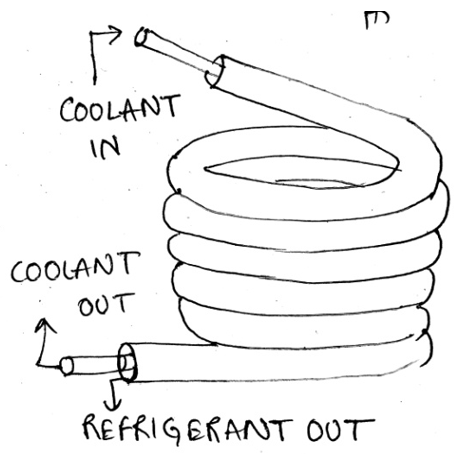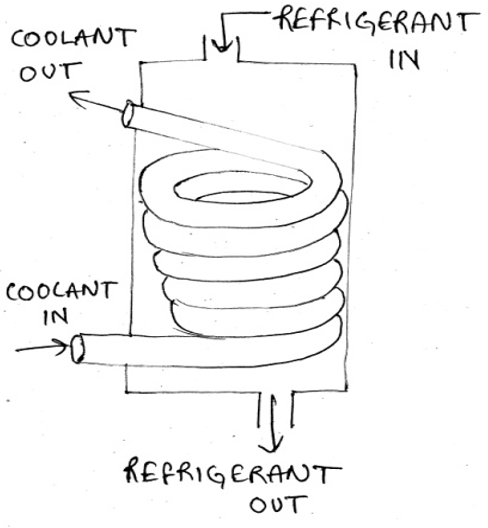Q.1
• a) Coil
• b) Shell
• c) Tube
• d) Annulus
Q.2
##### Recognize the following water cooled condenser.• a) Shell tube
• b) Double tube condenser
• c) Tube coil
• d) Shell coil
Q.3
##### Recognize the following water cooled condenser.• a) Shell and Tube condenser
• b) Double tube condenser
• c) Shell and coil type condenser
• d) Plate-type condenser
Q.4
##### In evaporative condensers, ___________ used to extract heat from the condensing refrigerant.
• a) Air is
• b) Both air and water are
• c) Water is
• d) Either air or water is
Q.5
##### Evaporative condensers combine the features of a _____________ in a single unit.
• a) Cooling tower and water-cooled condenser
• b) Falling tower and water-cooled condenser
• c) Cooling tower and Air condenser
• d) Rising film tower and water-cooled condenser
Q.6
##### Evaporative condensers are used in places where water is _________
• a) Scarce
• b) Plenty
• c) Moderately available
• d) Sufficient
Q.7
##### What is the meaning of heat rejection ratio (HRR) of a condenser?
• a) Ratio of heat rejected to the heat absorbed
• b) Ratio of heat absorbed to the heat rejected
• c) Ratio of heat required to the heat lost
• d) Ratio of heat wasted in a cycle to the heat absorbed
Q.8
##### What is the relation between heat rejection ratio (HRR) and Coefficient of performance (COP)?
• a) HRR = 1 + 1/COP
• b) HRR = 1+ COP
• c) HRR = COP/2
• d) HRR = 1/COP
Q.9
##### What is the equation for Coefficient of performance of a condenser?
• a) COP = Qe/Qc
• b) COP = We/Qc
• c) COP = Wc/Qe
• d) COP = Qe/Wc
Q.10
• a) 2-3’-3-4
• b) 3’-3
• c) 3-4
• d) 2-3’-3
Q.11
• a) True
• b) False